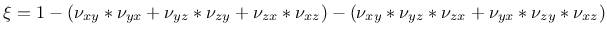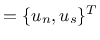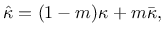## Bingham fluid - BinghamFluid

Constitutive model of Bingham fluid. This is a constitutive model of non-Newtonian type. The model parameters are summarized in Tab. 63.

In the Bingham model the flow is characterized by following constitutive equation(276)(277)

whereis the shear stress applied to material, is the shear stress measure, is the shear rate,is the yield stress, and is the plastic viscosity. The parameters for the model can be in general determined using two possibilities: (i) stress controlled rheometer, when the stress is applied to material and shear rate is measured, and (ii) shear rate controlled rheometer, where concrete is sheared and stress is measured. However, most of the widely used tests are unsatisfactory in the sense, that they measure only one parameter. These one-factor tests include slump test, penetrating rod test, and Ve-Be test. Recently, some tests providing two parameters on output have been designed (BTRHEOM, IBB, and BML rheometers). Also a refined version of the standard slump test has been developed for estimating yield stress and plastic viscosity. The test is based on measuring the time necessary for the upper surface of the concrete cone in the slump to fall a distance 100 mm. Semi-empirical models are then proposed for estimating yield stress and viscosity based on measured results. The advantage is, that this test does not require any special equipment, provided that the one for the standard version is available.

In order to avoid numerical difficulties caused by the existence of the sharp angle in material model response at , the numerical implementation uses following smoothed relation for viscosity

 (278)

whereis so called stress growth parameter. The higher value of parameter, the closer approximation of the original constitutive equation (276) is obtained.

Table 63: Bingham Fluid material - summary.
 Description Bingham fluid material Record Format BinghamFluid num(in) # d(rn) # mu0(rn) # tau0(rn) # Parameters - num material model number - d material density - mu0 viscosity - tau0 Yield stress Supported modes 2d, 3d flow

Borek Patzak
2018-01-02